•Gurobi Staff

Hi Dilip,

You can reformulate the min-max objective by introducing a single additional continuous variable z, and then move the tour distance calculation to a set of constraints, i.e.:

$$\min z$$

$$\sum_{i=1}^n \sum_{j=1}^n d_{ij} x_{ijk} \le z \qquad \forall k$$

In an optimal solution, the value of variable z will always correspond to the maximum of all tour distances.

Best regards,
Mario

•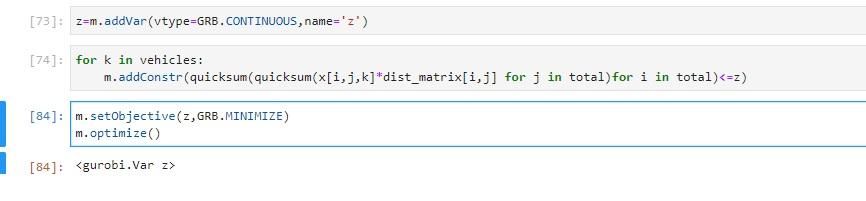Dear Mario,

Thanks for your suggestion i tried like you said in the figure. Still I am getting the model infeasible.  Did I formulate it correctly?

Hoping to hear from you soon.

Yours sincerely,

Dilip

•Gurobi Staff

Hi Dilip,

Your code for this particular model part looks correct, although you might write the quicksum in a more efficient way:

m.addConstr(quicksum(x[i, j, k]*dist_matrix[i,j] for i in total for j in total) <= z)

I guess the infeasibility comes from somewhere else.
Usually, I debug models by looking at the model file that is written with

m.write("model.lp")

Best regards,
Mario

•Hi Mario,

Thank you so much for your suggestion. actually this was the model I was modelling.

n=7
customers=[1,2,3,4,5,6]total=[0,1,2,3,4,5,6]
vehicles=[x for x in range(no_of_vehicles)]vehicles=[0,1,2]
for i in range(n):    for j in range(n):        dist_matrix[i, j] = np.sqrt((coordinates[i] - coordinates[j]) ** 2 + (coordinates[i] - coordinates[j]) ** 2)        if i==j:            dist_matrix[i,j]=M        continue
dist_matrix=
array([[1.00000000e+06, 3.16227766e+02, 2.82842712e+02, 0.00000000e+00,
4.00000000e+02, 2.82842712e+02, 6.00000000e+02],
[3.16227766e+02, 1.00000000e+06, 1.41421356e+02, 3.16227766e+02,
1.41421356e+02, 3.16227766e+02, 3.16227766e+02],
[2.82842712e+02, 1.41421356e+02, 1.00000000e+06, 2.82842712e+02,
2.82842712e+02, 4.00000000e+02, 4.47213595e+02],
[0.00000000e+00, 3.16227766e+02, 2.82842712e+02, 1.00000000e+06,
4.00000000e+02, 2.82842712e+02, 6.00000000e+02],
[4.00000000e+02, 1.41421356e+02, 2.82842712e+02, 4.00000000e+02,
1.00000000e+06, 2.82842712e+02, 2.00000000e+02],
[2.82842712e+02, 3.16227766e+02, 4.00000000e+02, 2.82842712e+02,
2.82842712e+02, 1.00000000e+06, 4.47213595e+02],
[6.00000000e+02, 3.16227766e+02, 4.47213595e+02, 6.00000000e+02,
2.00000000e+02, 4.47213595e+02, 1.00000000e+06]])
##first decision variablefor i in total:    for j in total:        for k in vehicles:            x[i,j,k] = m.addVar(vtype=GRB.BINARY, name="x%d,%d,%d" % (i, j,k))m.update()
## 2nd decision variable (equation 12)y={}for i in total:    for k in vehicles:        y[i,k] = m.addVar(vtype=GRB.BINARY, name="y%d,%d" % (i,k))m.update()
##non negative interger decision variable to remove sub toursf_vars={}for i in total:    for j in total:        for k in vehicles:            f_vars[i,j,k]=m.addVar(lb=0,vtype=gurobipy.GRB.INTEGER)m.update()
##equation 2m.addConstr(gurobipy.quicksum(gurobipy.quicksum(x[1,j,k] for j in customers)for k in vehicles)==no_of_vehicles)
##equation 3m.addConstr(gurobipy.quicksum(gurobipy.quicksum(x[h,1,k] for h in customers)for k in vehicles)==no_of_vehicles)
##equaton 4for i in total:    m.addConstr(gurobipy.quicksum(y[i,k] for k in vehicles)==1)
## equation 5for i in total:    for k in vehicles:        m.addConstr(gurobipy.quicksum(x[h,i,k] for h in total)==y[i,k])
##equation 6for i in total:    for k in vehicles:        m.addConstr(gurobipy.quicksum(x[i,j,k] for j in total)==y[i,k])
##equation 7for i in total:    for j in total:        for k in vehicles:            m.addConstr(f_vars[i,j,k]<=(n-1)*x[i,j,k])
##equation 8m.addConstr(gurobipy.quicksum(gurobipy.quicksum(f_vars[i,1,k]for i in total)for k in vehicles)==n-1)
##equation 9for i in total:    for k in vehicles:        m.addConstr(f_vars[1,j,k]==0)
##equation 10for i in total:    for k in vehicles:        m.addConstr(gurobipy.quicksum(f_vars[i,j,k] for j in total)-gurobipy.quicksum(f_vars[h,i,k] for h in total)==y[i,k])
z=m.addVar(vtype=GRB.CONTINUOUS,name='z')for k in vehicles:    m.addConstr(quicksum(quicksum(x[i,j,k]*dist_matrix[i,j] for j in total)for i in total)==z)
m.setObjective(z,GRB.MINIMIZE)m.optimize()m.write("model.lp")

So I wrote code for the photos attached in this comment and the previous one. So I did as you said but still the model is infeasible.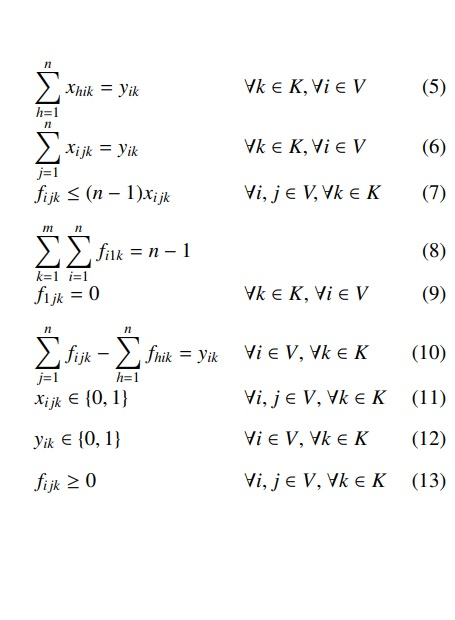I checked the model.lp file as i am new i just found the constraints that are there but no any errors signs on it.I have attached the model.lp file too.
Hoping for your some valuable suggestions
Yours sincerely,
•Gurobi Staff

Hi Dilip,

I can spot two issues:

1. I think your depot is 0 according to the definition of "customers" and "total", but in your formulation you assume customer 1 to be the depot.
2. In the last set of constraints you have "== z" but it should be "<= z".

Best regards,
Mario

•Hi  Mario,

Thank you so much for you correction. I assumed like this, I have the list of depot and customers stored in total .. So I assumed '0' as the depot and customers as [1,2,3,4,5,6].  As in equation 3 and 2 since it starts from j=2 and h=2 it means that they are for the customers right? .So in the above formulation they have cities V={1,2,3,4,5,6,7} and depot as 1, but I took V={0,1,2,3,4,5,6}. So I think I did the same as the author assumed. I can't find where I assumed 1 to be the depot. I searched it in my formulation.

Yours sincerely,

Dilip Maharjan

•Hi Mario,

thank you again for your help

Actually The models that I was able to solve were as follow.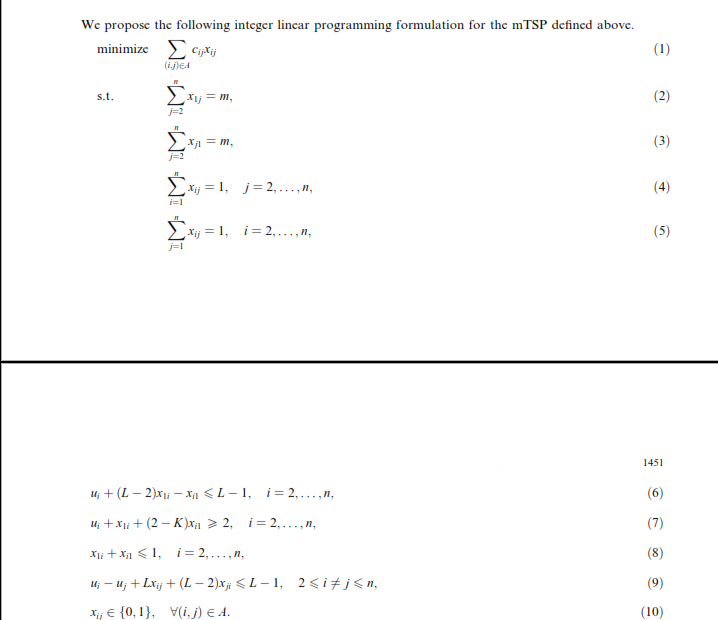so I was able to minimize the cost but how can i minmax in this model above and below.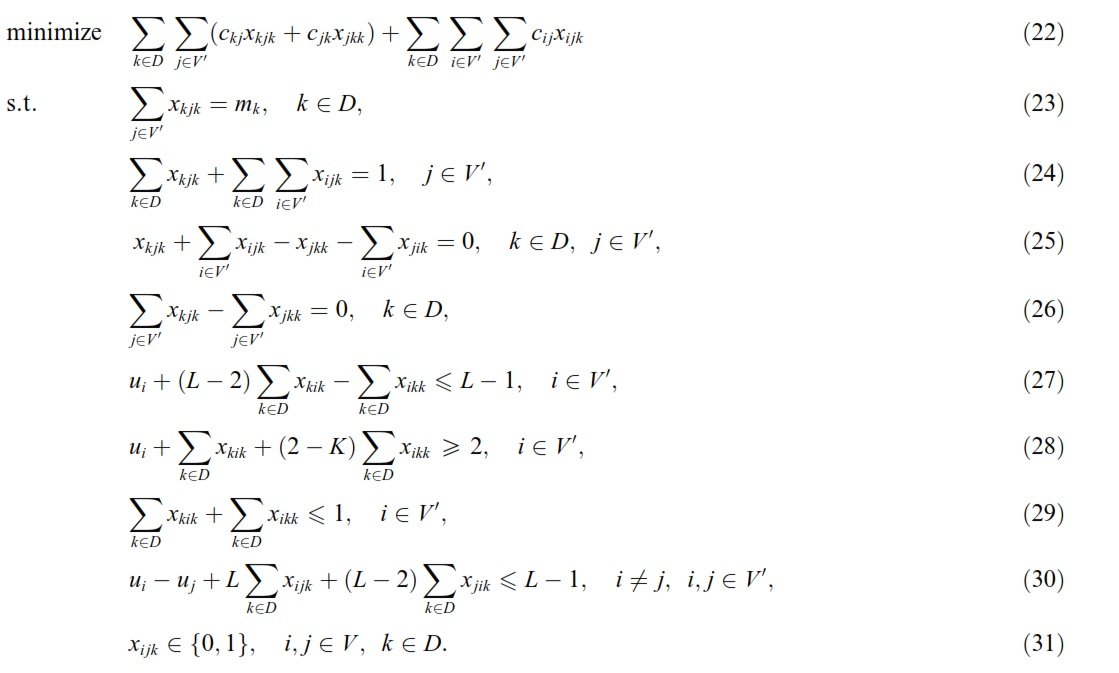I was able to minimize and solve both of them but , how can i get min max in these two? .Do I need to change the model completely or add some new constraints and variables ?

Yours sincerely,

Dilip Maharjan

•Hi Mario I ran my both those model by doing this

z=m.addVar(vtype=GRB.CONTINUOUS,name='z')m.addConstr(gp.quicksum(gp.quicksum(dist_dep_cus[k,j]*x[k,j,k]+dist_dep_cus[k,j]*x[j,k,k] for j in customers)for k in Depot )+gp.quicksum(gp.quicksum(gp.quicksum(dist_cus_cus[i,j]*x[i,j,k] for j in customers)for i in customers) for k in Depot)<=z)m.setObjective(z,GRB.MINIMIZE)

the above one was for the second and the down was for the first one

z=m.addVar(vtype=GRB.CONTINUOUS,name='z')m.addConstr(quicksum(quicksum(x[(i, j)]*dist_matrix[(i, j)] for j in range(n)) for i in range(n))<=z)m.setObjective(z,GRB.MINIMIZE)m.optimize()

Both the model ran doing this

I think now I did the correct thing right? :)

Anyways thank you so much for your help and suggestions

Yours sincerely,

Dilip Maharjan

•Gurobi Staff

Hi Dilip,

I am not sure if I can follow your last messages. Do you still have problems?

Best regards,
Mario

•Hello Mario,

Actually I just wanted to be sure how to make min max in the following objective function. I could minimize the both the models below. but I am not sure how to make it min max. Can you suggest me something about it?2nd model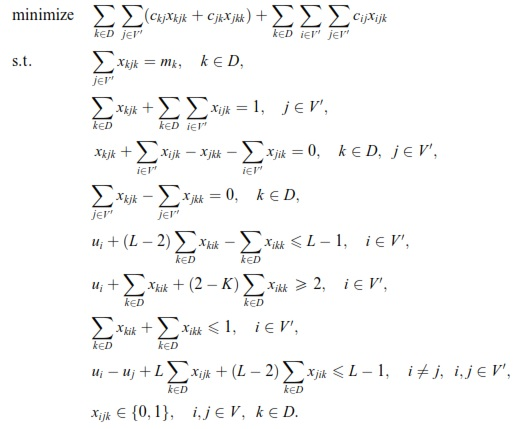Yours sincerely,

Dilip Maharjan

•Gurobi Staff

Hi Dilip,

We already discussed how you can model a min-max objective function, see the messages above.
So, could you be a bit more precise about what does not work with the approach I suggested?

Best regards
Mario

•Hi Mario ,

In the last post i have posted two models of mTSP case .SO I wanted to change the objective function in both case into min-max objective function type. So as you suggested I did following.

I did following for the 2nd model.

z=m.addVar(vtype=GRB.CONTINUOUS,name='z')m.addConstr(gp.quicksum(gp.quicksum(dist_dep_cus[k,j]*x[k,j,k]+dist_dep_cus[k,j]*x[j,k,k] for j in customers)for k in Depot )+gp.quicksum(gp.quicksum(gp.quicksum(dist_cus_cus[i,j]*x[i,j,k] for j in customers)for i in customers) for k in Depot)<=z)m.setObjective(z,GRB.MINIMIZE)

and for the  first model too

z=m.addVar(vtype=GRB.CONTINUOUS,name='z')m.addConstr(quicksum(quicksum(x[(i, j)]*dist_matrix[(i, j)] for j in range(n)) for i in range(n))<=z)m.setObjective(z,GRB.MINIMIZE)m.optimize()

So I want to be sure what I did  is correct or not . I just wanted to be sure on it.

Yours sincerely,

Dilip Maharjan

•Gurobi Staff

Hi Dilip,

Did you try it and analyze the solutions?

2nd model: I do not think that it works since you just added a single constraint, instead of one constraint for each vehicle k. 12 days ago you already sent me a code snippet that looked correct (as I replied). Now, you seem to have changed it again.

1st model: Here, you have no vehicle index k, so it is not straight-forward to minimize the maximal route distance. If you really need to avoid the vehicle index k, then you need to introduce additional model parts to keep track of the total distance up to some customer i, similar to the variables u_i to keep track of a customer's position in a route.

Best regards,
Mario

•Hi Mario,

I was able to solve the 2nd model , I am trying to convert the first model in case of minmax and I wrote the model in following ways

for the equation 2nd of the 1st model

for k in range (no_of_vehicles):    m.addConstr(gurobipy.quicksum(x[0, i,k] for i in range(1, n)) == no_of_vehicles)

for 3rd equation

for k in range (no_of_vehicles):    m.addConstr(gurobipy.quicksum(x[i, 0,k] for i in range(1, n)) == no_of_vehicles)

for 4th equation

for k in range(no_of_vehicles):    for i in range(1, n):        m.addConstr(gurobipy.quicksum(x[i, j,k] for j in range(n) if i != j) == 1)

for 5th equation

for k in range(no_of_vehicles):    for j in range(1, n):        m.addConstr(gurobipy.quicksum(x[i, j,k] for i in range(n) if i != j) == 1)

for 6th equation

for  k in range(no_of_vehicles):    for i in range(1, n):        m.addConstr(u_vars[i] + (L - 2) * x[0, i,k] - x[i, 0,k] <= L - 1)

for equation 7

for k in range(no_of_vehicles):    for i in range(1, n):        m.addConstr(u_vars[i] + x[0, i,k] + (2 - K) * x[i, 0,k] >= 2)

for equation 8

for k in range(no_of_vehicles):    for i in range(1, n):        m.addConstr(x[0, i,k]+ x[i, 0,k]<= 1)

for equation 9

for k in range(no_of_vehicles):    for i in range(1, n):        for j in range(1, n):            if i != j:                m.addConstr(u_vars[i] - u_vars[j] + L * x[i, j,k] + (L - 2) * x[j, i,k] <= L - 1)
z=m.addVar(vtype=GRB.CONTINUOUS,name='z')for k in range(no_of_vehicles):    m.addConstr(quicksum(quicksum(x[(i, j,k)]*dist_matrix[(i, j)] for j in range(n)) for i in range(n))<=z)
m.setObjective(z,GRB.MINIMIZE)m.optimize()

So I added the vehicle index k but I am not so confident about the changes to be made in the constraints while adding the vehicle index k . I ran the model but I got the attributes like below.

    Variable            X
-------------------------
x0,1,0            1
x0,1,1            1
x0,1,2            1
x0,6,0            1
x0,6,1            1
x0,6,2            1
x0,7,0            1
x0,7,1            1
x0,7,2            1
x1,10,0            1
x1,10,1            1
x1,10,2            1
x2,0,0            1
x2,0,1            1
x2,0,2            1
x3,5,0            1
x3,5,1            1
x3,5,2            1
x4,9,0            1
x4,9,1            1
x4,9,2            1
x5,0,0            1
x5,0,1            1
x5,0,2            1
x6,4,0            1
x6,4,1            1
x6,4,2            1
x7,8,0            1
x7,8,1            1
x7,8,2            1
x8,2,0            1
x8,2,1            1
x8,2,2            1
x9,0,0            1
x9,0,1            1
x9,0,2            1
x10,3,0            1
x10,3,1            1
x10,3,2            1
u1            1
u2            3
u3            3
u4            2
u5            4
u6            1
u7            1
u8            2
u9            3
u10            2
z      5566.08 

I think  I made wrong while adding the index for each vehicle in the constraints. Can you guide me a little with the changes to be made while adding the vehicle index?

Yours sincerely,

Dilip Maharjan

•Hello Mario,

In the previous post I think I rebuilt the model incorrectly while putting the index of vehicle. So I have rebuilt a new mathematical model for the model 1 to work it correctly in terms of min max as below picture.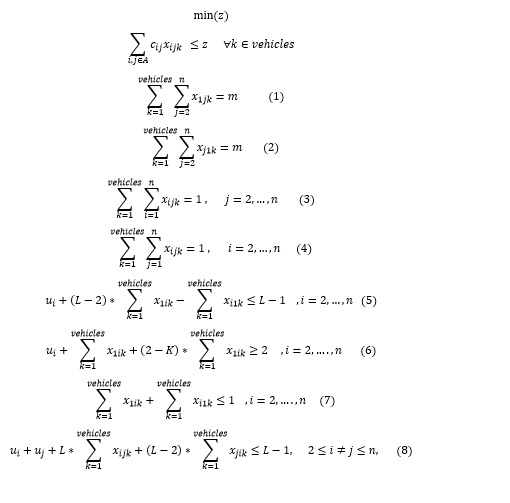the vehicles in the above model means total number of vehicles.

So is the model I built correct ?.

Hoping for your kind consideration and hoping to hear from you soon

Yours sincerely,

Dilip Maharjan

•Gurobi Staff

Hi Dilip,

Yes, this looks correct.

Mario

•Hi Mario I ran  the model shown above as below:

coordinates=[[565.685424949238, 424.26406871192853],
[141.4213562373095, 424.26406871192853],
[565.685424949238, 848.5281374238571],
[848.5281374238571, 0.0],
[141.4213562373095, 848.5281374238571],
[565.685424949238, 282.842712474619],
[0.0, 141.4213562373095],
[141.4213562373095, 565.685424949238],
[707.1067811865476, 0.0],
[707.1067811865476, 565.685424949238]]
coordinates=[[0,0]]+coordinates  ## I added depot here
M=1000*1000  ## just a big value
n = len(coordinates)
L=n ##parameter that  describes the maximum number of cities to be visited
coordinates=np.array(coordinates)
no_of_vehicles=2  ## number of vehicles
dist_matrix = np.empty([n, n])  ##  Distance matrix initialization
m = Model("MVRP")

Distance matrix calculation below

for i in range(n):    for j in range(n):        dist_matrix[i, j] = np.sqrt((coordinates[i] - coordinates[j]) ** 2 + (coordinates[i] - coordinates[j]) ** 2)        if i==j:            dist_matrix[i,j]=M        continue
x={}    #decision variable initialization
for i in range(n):    for j in range(n):        for k in range(no_of_vehicles):            x[i, j,k] = m.addVar(vtype=GRB.BINARY, name="x%d,%d,%d" % (i, j,k))m.update()

integer variable initialization

u_vars = {}for i in range(n):    u_vars[i] = m.addVar(lb=0, ub=L, vtype=gurobipy.GRB.INTEGER, name='u' + str(i))m.update()

#constraint 1

m.addConstr(gp.quicksum(gp.quicksum(x[0,j,k] for j in range(1,n))for k in range(no_of_vehicles))==no_of_vehicles)

Constraint 2

m.addConstr(gp.quicksum(gp.quicksum(x[j,0,k] for j in range(1,n))for k in range(no_of_vehicles))==no_of_vehicles)

Constraint 3

for i in range(1, n):    m.addConstr(gp.quicksum(gp.quicksum(x[i, j,k] for j in range(n))for k in range(no_of_vehicles)) == 1)

constraint 4

for j in range(1, n):    m.addConstr(gp.quicksum(gurobipy.quicksum(x[i, j,k] for i in range(n))for k in range(no_of_vehicles)) == 1)

constraint5

for i in range(1,n):    m.addConstr(u_vars[i]+(L-2)*gp.quicksum(x[0,i,k] for k in range(no_of_vehicles))-gp.quicksum(x[i,0,k] for k in range(no_of_vehicles))<=L-1)

constraint 6

for i in range(1,n):    m.addConstr(u_vars[i]+gp.quicksum(x[0,i,k] for k in range(no_of_vehicles))+(2-K)*gp.quicksum(x[i,0,k] for k in range(no_of_vehicles))>=2)

Constraint 7

for i in range(1,n):    m.addConstr(gp.quicksum(x[0,i,k] for k in range(no_of_vehicles))+gp.quicksum(x[i,0,k] for k in range(no_of_vehicles))<=1)

Constraint 8

for i in range(1,n):    for j in range(1,n):        if i !=j:            m.addConstr(u_vars[i]-u_vars[j]+L*gp.quicksum(x[i,j,k] for k in range(no_of_vehicles))+(L-2)*gp.quicksum(x[j,i,k] for k in range (no_of_vehicles))<=L-1)

Constraint last

z=m.addVar(vtype=GRB.CONTINUOUS,name='z')for k in range(no_of_vehicles):    m.addConstr(quicksum(quicksum(x[(i, j,k)]*dist_matrix[(i, j)] for j in range(n)) for i in range(n))<=z)
m.setObjective(z,GRB.MINIMIZE)m.optimize()

The model ran and gave optimized value But the attribute X was like this below:

m.printAttr('X')
   Variable            X
-------------------------
x0,7,1            1
x0,8,0            1
x1,0,0            1
x2,6,0            1
x3,10,1            1
x4,9,1            1
x5,3,1            1
x6,4,1            1
x7,2,0            1
x8,5,0            1
x9,0,1            1
x10,1,1            1
u1            5
u2            2
u3            3
u4            4
u5            2
u6            3
u7            1
u8            1
u9            5
u10            4
z      2336.49 

so if we analyze the routes its like this Route1 is 0-7-2-6-4-9-0 and Route 2  is 0-8-5-3-10-1-0. The route is correct but in the both route vehicles 0 and 1 are there. What I mean is the vehicle 1 moves from 0-7 but from 7-2 vehicle 0 moves and 2-6 its vehicle 0 whereas from 6-4 its vehicle 1 ,4-9 its vehicle` and 9-0 its vehicle 1 again. Similarly in another route also there are two vehilces.

So in my model am I missing any constraint so that each route would be covered by just 1 vehicle. Can you suggest me any constraint so that the model would run perfectly,